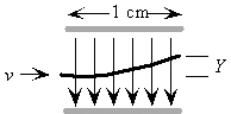# College Physics Study Set 7

Physics & Astronomy

## Quiz 16 : Electrical Energy and CapacitanceStudy FlashcardsLooking for Introductory Physics Homework Help?

## Quiz 16 :Electrical Energy and Capacitance

Question TypeA proton (+1.6 * 10-19 C) moves 10 cm along the direction of an electric field of strength 3.0 N/C.The electrical potential difference between the proton's initial and ending points is:
Free
Multiple Choice

B

Tags
Choose question tagAn electron with velocity v = 1.0 * 106 m/s is sent between the plates of a capacitor where the electric field is E = 500 V/m.If the distance the electron travels through the field is 1.0 cm, how far is it deviated (Y) in its path when it emerges from the electric field? (me = 9.1 * 10-31 kg, e = 1.6 * 10-19 C)Free
Multiple Choice

B

Tags
Choose question tagIf the distance between two isolated parallel plates that are oppositely charged is doubled, the electric field between the plates is essentially unchanged.However, the:
Free
Multiple Choice

A

Tags
Choose question tagAn ion is released from rest and moves due to the force from an electric field from a position in the field having a potential of 14 V to a position having a potential of 8 V.The ion:
Multiple Choice
Tags
Choose question tagAn electron is released from rest at the negative plate of a parallel-plate capacitor.If the distance across the plate is 5.0 mm and the potential difference across the plate is 5.0 V, with what velocity does the electron hit the positive plate? (me = 9.1 * 10-31 kg, e = 1.6 * 10-19 C)
Multiple Choice
Tags
Choose question tagThe quantity of electrical potential, the volt, is dimensionally equivalent to:
Multiple Choice
Tags
Choose question tagA uniform electric field, with a magnitude of 600 N/C, is directed parallel to the positive x-axis. If the potential at x = 3.0 m is 1 000 V, what is the change in potential energy of a proton as it moves from x = 3.0 m to x = 1.0 m? (qp = 1.6 * 10-19 C)
Multiple Choice
Tags
Choose question tagIf an electron is accelerated from rest through a potential difference of 1 200 V, find its approximate velocity at the end of this process.(e = 1.6 * 10-19 C; me = 9.1 * 10-31 kg)
Multiple Choice
Tags
Choose question tagA proton (+1.6 * 10-19 C) moves 10 cm on a path in the direction of a uniform electric field of strength 3.0 N/C.How much work is done on the proton by the electrical field?
Multiple Choice
Tags
Choose question tagWhich of the following characteristics are held in common by both gravitational and electrostatic forces when dealing with either point masses or charges?
Multiple Choice
Tags
Choose question tagThe unit of electrical potential, the volt, is dimensionally equivalent to:
Multiple Choice
Tags
Choose question tagA 9.0-V battery is connected between two parallel metal plates 4.0 mm apart.What is the magnitude of the electric field between the plates?
Multiple Choice
Tags
Choose question tagAn electron (charge -1.6 * 10-19 C) moves on a path perpendicular to the direction of a uniform electric field of strength 3.0 N/C.How much work is done on the electron as it moves 15 cm?
Multiple Choice
Tags
Choose question tagIn which case does an electric field do positive work on a charged particle?
Multiple Choice
Tags
Choose question tagA free electron is in an electric field.With respect to the field, it experiences a force acting:
Multiple Choice
Tags
Choose question tagA uniform electric field, with a magnitude of 600 N/C, is directed parallel to the positive x-axis.If the potential at x = 3.0 m is 1 000 V, what is the potential at x = 1.0 m?
Multiple Choice
Tags
Choose question tagAn electron in a cathode ray tube is accelerated through a potential difference of 5.0 kV.What kinetic energy does the electron gain in the process? (e = 1.6 * 10-19 C)
Multiple Choice
Tags
Choose question tagIf the distance between two negative point charges is increased by a factor of three, the resultant potential energy is what factor times the initial potential energy?
Multiple Choice
Tags
Choose question tagFour point charges are on the rim of a circle of radius 10 cm.The charges are (in mC) +0.50, +1.5, -1.0, -0.50.If the electrical potential at the circle's center due to the +0.5 charge alone is 4.5 * 104 V, what is the total potential at the center due to the four charges combined?
Multiple Choice